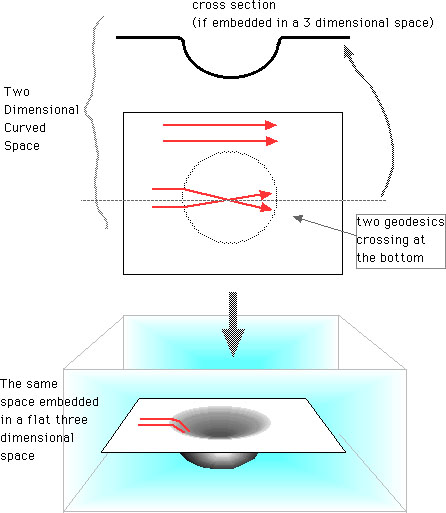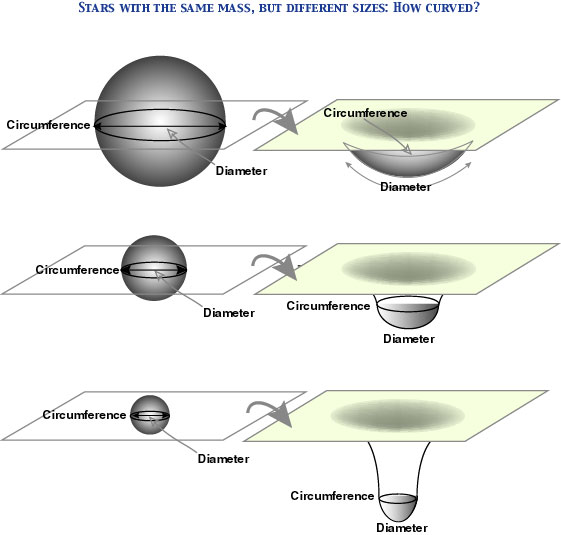Phil. Space and Time

Embedding Diagram

Embedding Diagram

It is hard for us to visualize a curved space or spacetime, especially if that space (spacetime) has three or more dimensions. However, physicists have invented a "trick" for visualizing a curved two-dimensional surface within an imaginary, flat, three-dimensional space, and that trick is called an "embedding diagram" (Misner et al 1973, 613ff; Thorn 1994, 129ff. öMö—1997, 112ff.). You already know the distinction of intrinsic and extrinsic curvature. For instance, the surface of a sphere is a curved two-dimensional surface, and its curvature can be identified by measurements within that surface; thus we can say that the surface has an intrinsic curvature. However, we can of course visualize it within a three-dimensional space, by regarding it as the surface of a sphere in a flat, three-dimensional Euclidean space. The embedding diagram utilizes this relation. But do not imagine a higher dimensional space used for embedding as something "real"; it is only a fiction for enabling us to visualize the curvature.

Suppose a simple two-dimensional space, which has a concavity in the middle, as in the figure. Gravity in general relativity is expressed by the curvature of spacetime. This means that light rays, or free particles go along a geodesic, a straightest line allowed by the curvature. Thus if two light rays (red lines) come to the concavity, they will cross at the bottom (because of the curvature of this part), and this can be expressed by a two-dimensional figure. However, the image is somehow poor, and a better visualization is to embed this space into a three-dimensional Euclidean space, and express its curvature as an extrinsic curvature of a two-dimensional surface within this 3-space. The result is an embedding diagram.The same trick can be applied to any two-dimensional surface in a curved 3-space or 4-space (spacetime). For instance, the equatorial plane crossing a star (spherical, without spin, and with a constant density) is a curved 2-space, and its embedding diagram is obtained as follows. For the sake of comparison, we consider stars with the same mass but with different sizes; you can see (intuitively) how the curvature of the equatorial plane (2-space) changes. On the left-hand side are pictures of physical (3-) space, and on the right-hand side are embedding diagrams of the equatorial plane (2-space). These embedding diagrams illustrate Schwarzshild's solution to the Einstein field equations. Such a diagram can be extended to a black hole too.[The figure adapted from Thorn (1994), Fig. 3.4 on page 132.]

References

Misner, C. W., Thorne, K. S., and Wheeler, J. A. (1973) Gravitation, Freeman, 1973.

Thorn, Kip S. (1994) Black Holes and Time Warps, Papermac, 1995. [–M–ó1997]